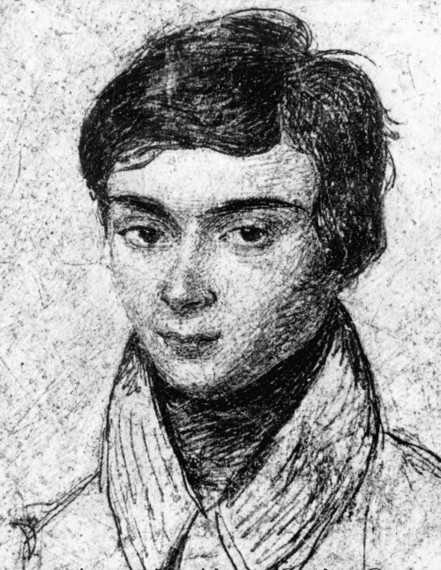# The Most Romantic Mathematician and His Language of Symmetry

On the morning of May 30, 1832, a single shot fired in a duel fatally wounded one of the most brilliant, and certainly the most romantic mathematician -- Évariste Galois. The following day, his last words to his weeping brother were: "Don't cry, I need all my courage to die at 20." Figure 1 shows Galois at about age 15, as drawn by a classmate.Figure 1. Galois in about 1826, drawn by a classmate. This image is in the public domain. It was possessed by Nathalie-Théodore Chantelot, his older sister, and her daughter Mrs. Guinard. The image was released by Paul Dupuy, École Normale Supérieure professor of history, with his article "La vie d'Évariste Galois," in 1896.

This young genius achieved nothing less than inventing the mathematical language that describes all the symmetries of the world. Whether one analyzes symmetries of shapes, in music, or in particle physics, the same formalism -- Group Theory -- applies.

What is a mathematical "group"? Take as an example the whole numbers, positive, negative and zero, and the simple operation of arithmetic addition. You'll notice the following properties:
1. When you add two whole numbers, you get another whole number. For example, 3 + 6 = 9; -7 + 15 = 8.
2. If you add three whole numbers, it doesn't matter how you group them. For example, (9 + 3) + 5 = 9 + (3 + 5).
3. There exists one whole number that when you add it to any other whole number, it leaves the latter unchanged. This is, of course, the number zero. For instance, 13 + 0 = 13.
4. For every whole number, there exists another whole number such, that if you add then together you get zero. For example: 8 + (-8) = 0; (-17) + 17 = 0.

Any collection of objects and an associated operation that satisfy these four conditions is called a group.

How are groups connected to symmetries? Let's take as a simple example the external bilateral symmetry of the human body (and assume that it is perfect). We can take as our group members the two transformations: I - the identity, which leaves the body unchanged. R - a reflection about a vertical plane. As the operation, we will simply apply transformations successively (and we'll denote that operation by "×"). So, for example, I × R = R, since if we first reflect, and then do nothing, the end result will be a reflection. R × R = I, because if you reflect twice, you leave the body as is.

You can easily convince yourself that these two transformations obey the four properties above, and therefore form a group.

It's hard to believe, but from these seemingly trivial concepts emerged a language that is the bread and butter of all the symmetries (and the notion of symmetry-breaking) in modern physics. Yes, even the roots to the idea of the existence of the Higgs particle!

Unfortunately for Galois himself, he did not live to see the fruits of his incredible invention. In the French tradition of "Cherchez La Femme," he fell in love with the wrong 17-year-old, and that sorrowful affair led to a miserable duel.

On the night before the duel, Galois made some last-minute corrections and commentaries to his mathematical papers (contrary to some popular myths, he did not formulate the entire theory that night). In one place, he wrote his most memorable quote: "Je n'ai pas le temps" -- "I have no time."